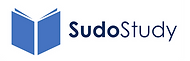top of page

#### Projectile Motion

Showing the independence of vertical and horizontal motion with a resulting parabolic path.

Anchor 1

### 1. Projectile Motion (part 1)

Don't worry - it's not real! This is just one example of projectile motion and a way that you can solve equations where an object is initially travelling horizontally. Remember the vertical component of velocity is independent of its horizontal component so it accelerates vertically but not horizontally.

Anchor 2

### 2. Projectile Motion (part 2)

Indirect fire (provided by the finest regiment in the British Army - the Royal Artillery) is the example I used in this video. When you have a projectile fired at at an angle (it initially has both horizontal and vertical components of velocity) it will follow a parabolic path. This video shows how you can solve such problems using simple 'suvat' equations.

Anchor 3

### 3. Projectile Motion (part 3)

This is a rather long example with a projectile fired at an angle from a raised platform. I show you how to break up the motion of the projectile into three sections then use suvat for both the horizontal and vertical components of velocity.bottom of page